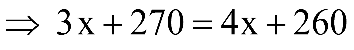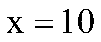A man engaged a servant on the condition that he would pay him Rs. 90 and  a turban after a service of one year. He served only for nine months and receive the turban and Rs.15. The price of the turban is.

# A man engaged a servant on the condition that he would pay him Rs. 90 and  a turban after a service of one year. He served only for nine months and receive the turban and Rs.15. The price of the turban is.

1. A
Rs 25
2. B
Rs 18.75
3. C
Rs. 10
4. D
Rs. 2.50

Fill Out the Form for Expert Academic Guidance!l

+91

Live ClassesBooksTest SeriesSelf Learning

Verify OTP Code (required)

### Solution:

Let the price of the turban be Rs. x
Then, total income for 12 months = Rs. (90 + x)
Income for 9 months## Related content

 Area of Square Area of Isosceles Triangle Pythagoras Theorem Triangle Formula Perimeter of Triangle Formula Area Formulae Volume of Cone Formula Matrices and Determinants_mathematics Critical Points Solved Examples Type of relations_mathematics+91

Live ClassesBooksTest SeriesSelf Learning

Verify OTP Code (required)